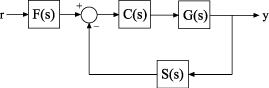Control System Model With Both Numeric and Tunable Components

This example shows how to create a tunable model of a control system that has both fixed plant and sensor dynamics and tunable control components.

Consider the control system of the following illustration.Suppose that the plant response is $G\left(s\right)=1/\left(s+1{\right)}^{2}$, and that the model of the sensor dynamics is $S\left(s\right)=5/\left(s+4\right)$. The controller $C$ is a tunable PID controller, and the prefilter $F=a/\left(s+a\right)$ is a low-pass filter with one tunable parameter, a.

Create models representing the plant and sensor dynamics. Because the plant and sensor dynamics are fixed, represent them using numeric LTI models.

G = zpk([],[-1,-1],1);
S = tf(5,[1 4]);

To model the tunable components, use Control Design Blocks. Create a tunable representation of the controller C.

C = tunablePID('C','PID');

C is a tunablePID object, which is a Control Design Block with a predefined proportional-integral-derivative (PID) structure.

Create a model of the filter $F=a/\left(s+a\right)$ with one tunable parameter.

a = realp('a',10);
F = tf(a,[1 a]);

a is a realp (real tunable parameter) object with initial value 10. Using a as a coefficient in tf creates the tunable genss model object F.

Interconnect the models to construct a model of the complete closed-loop response from r to y.

T = feedback(G*C,S)*F
T =

Generalized continuous-time state-space model with 1 outputs, 1 inputs, 5 states, and the following blocks:
C: Tunable PID controller, 1 occurrences.
a: Scalar parameter, 2 occurrences.

Type "ss(T)" to see the current value, "get(T)" to see all properties, and "T.Blocks" to interact with the blocks.

T is a genss model object. In contrast to an aggregate model formed by connecting only numeric LTI models, T keeps track of the tunable elements of the control system. The tunable elements are stored in the Blocks property of the genss model object. Examine the tunable elements of T.

T.Blocks
ans = struct with fields:
C: [1x1 tunablePID]
a: [1x1 realp]

When you create a genss model of a control system that has tunable components, you can use tuning commands such as systune to tune the free parameters to meet design requirements you specify.